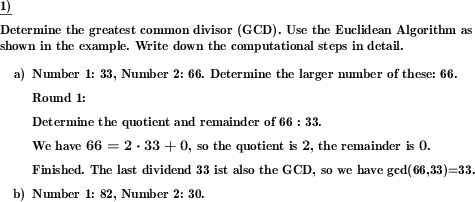Custom math worksheets at your fingertips# Details for problem "GCD computation with the Euclidean Algorithm"

Quickname: 2001

Elementary School, Primary School, Junior High School, Middle School, High School.

## Summary

Compute the GCD step by step with the Euclidian Algorithm.

## Example## Description

The tasks is to perform a detailed calculation of the largest common divisor (GCD) of two whole numbers with the Euclidean algorithm. The number space from which the numbers are chosen is adjustable. Also the number of problems can be selected.

To perform the computation, the following steps are repeated in rounds according to the algorithm.

1. Determine the quotient and remainder of the larger and the smaller number.
2. If the remainder is zero, the GCD has been determined. It is the last quotient.
3. Select the divisor of this round as the dividend for the next round. The divisor for the next round will be the remainder of this round.
4. Repeat from step one with the new dividend and divisor.

The first problem can be configured as a sample problem, which will be presented with the solution.

## Free worksheets and solution sheets with answers for download

Download free printable worksheets for this math problem here. The worksheet contains the problems only, the solution sheet includes the answers. Just click on the respective link.

•Worksheet 1Solution sheet with answers
•Worksheet 2Solution sheet with answers
•Worksheet 3Solution sheet with answers

If you can not see the solution sheets for download, they may be filtered out by an ad blocker that you may have installed. If this is the case, please allow ads for this page and reload the page. The solution sheets will then reappear.

• Do these sample worksheets do not really fit?
• Do you need more math worksheets, with a different level of difficulty?
• Would you like to combine different problems on a worksheet and adjust them to your needs?
• As a teacher, you can put together your own worksheets using the automatically generated math problems provided.
With a free initial credit, you can start creating your own math worksheets in a few minutes.

You can try it for free! Register here, to create custom worksheets now!

## Customization options for this problem

Parameter
Possible values
Number of problems
1, 2, 3, 4, 5, 6, 7, 8, 9, 10
Number range
50, 80, 100, 200, 500, 1000
Sample problem with answer
Yes, No

## Similar problems

Remark
Description
Name and direct link
Simpler approach to gcd: compare lists of factors to determine gcd
For two given numbers, the gcd and lcm are determined by comparing lists of multiples or divisors

## dw-Math worksheet templates that do contain this problem

Name
Title
Description
Greatest Common Divisor
Two ways to determine the gcd of two numbers.You may pass on the worksheets offered for download on this page to others, but not modify or sell them.